# Cramer’s Rule Calculator – 2 and 3 Equations System

## Cramer’s Rule & Calculator for Linear Circuit Analysis | Step by Step with Solved Examples

Today, we are going to share another simple but powerful circuit analysis technique which is known as “Cramer’s Rule“.
Update: We have added Online Cramer’s Rule Calculator where you can solve two equations system as well as three equations system. Check both Cramer’s rule calculator in both sections of the post. ThanksBelow is the Step by Step tutorial of solved examples, which elaborates that how to solve a complex electric circuit and network by Cramer’s rule.

### Cramer’s Rule Calculator for 2×2 (Two Equations System)

Cramer’s Rule Calculator 2 x 2 (2 Equations System):
 x + y = x + y = x = y =

### Finding Two Variables by Cramer’s Rule:

Example 1:
(In our case, the unknown values are two currents which are i1 and i2) by Cramer’s Rule. Now, let’s begin.

As shown below, this is a simple electric circuit and we are going to solve it by Cramer’s rule.
Solution:
First, rearrange the circuit with proper labels (As two of 5Ω resistors are in series, so, we will replace it with 10Ω.Apply Mesh Analysis and Simplify by Cramer’s Rule to find the unknown values of Currents i1 and i2.
Now, we will write the KVL equations of unknown values for the given circuit
Apply KVL on Mesh (1).
6 = 14i1 + 10(i1i2)
6 = 24i1 – 10i2 ….. → Eq (1)
Also, Apply KVL on Mesh (2).
-5 = 10 i2 + 10(i2 i1)
-5 = – 10 i1 + 20 i2 ….. → Eq (2)
Here, we got two equations, i.e.
24 i1 – 10 i2  =   6
– 10 i1 + 20 i2 = -5

Now, we will solve these two equations by Cramer’s rule to find the unknown values (of currents), which are i1 and i2.

Solving by Cramer’s rule:
Step 1:
First of all, write the above equations in the matrix form. i.e.Step 2:
Now, write the coefficient matrix of the above equations and call it ∆. Make sure it is square, i.e. Number of Rows x Number of Columns. In the above case, it has 2 rows and 2 columns.Step 3:
Now find the determinant |∆| of the coefficient matrix ∆ by the following method. (The given fig below will help you to do so.)
Click image to enlarge
According the above fig. the final step would be like this.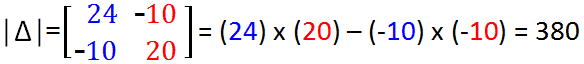Step 4:
Now find the coefficient determinant of Δ1 by the same method as mentioned above, but replace the first column of Δ with the “Answer column” (If you didn’t get the point of the answer column, See the fig in step 2 above or check the infographics at the end of the example of just refer to the second example below, where we did the same to find Δ1) which is,Step 5:
Now find the coefficient determinant of Δ2, just replace the second column with the “Answer Column” which is,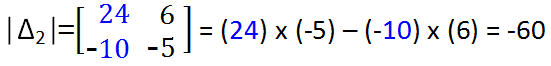Step 6:
As Cramer’s rule tells that i1= Δ1 / Δ and i2 = Δ2 / Δ.
Now, Find i1 and i2 by Cramer’s rule.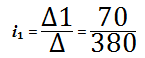i1= 0.184.2 A or 184.2 mA

And,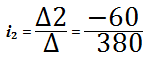i2 = 0.157.9 A or 157.9 mA

Below is the infographics summary of Cramer’s rule to determine the two variables or unknown values.
Ok, it was easy… Now, How about 3 variables…. Let’s try to solve 3 variables linear equations with the help of Cramer’s rule.

### Finding Three Variables by Cramer’s Rule:

(In our case, these unknown values are three currents which are i1, i2 and i3) by Cramer’s Rule. Now, let’s begin.

### Cramer’s Rule Calculator for 3×3 (Three Equations System)

Cramer’s Rule Calculator 3 x 3 (3 Equations Systems ):
 x + y + z = x + y + z = x + y + z = x = y = z =

Example 2:
Use Mesh Analysis to determine the three mesh currents in the circuit below. Use Cramer’s rule for simplification.
First of all, apply the KVL on each mesh one by one, and write its equations.
-7+1(i1i2) +6+2(i1i3) = 0
1(i2 i1) + 2i2 + 3(i2 i3) = 0
2(i3 i1) – 6+3(i3 i2) +1i3 = 0
Simplifying,
3i1 i2 – 2i3 = 1                    … Eq….. (1)
– i1 + 6i2 – 3i3 = 0                    … Eq….. (2)
-2i1 – 3i2 + 6i3 = 6                    … Eq….. (3)

Now, write the above equations in the matrix form.
3i1i2– 2i3 = 1
–i1+ 6i2– 3i3 = 0
-2i1– 3i2+ 6i3 = 6Now, we will find the coefficient determinant of ∆. How will we do that? Just check the fig below for better explanation.
So the full step is shown below.∆ = +3 (6 x 6) – (- 3 x –3) – (-1 (-1 x 6)-(-2 x –3) + (-2 (-1 x –3) – (-2 x 6)
= 81 -12 -30 = 39

So, here is the full step to find ∆1. Here, we replaced the “Blue Guys” in the first column with “Black Guys” :).= +1(36-9) – (1[0+18]) –2(0-36)
= 27 + 18 + 72
1 = 117

Again, find the ∆2 with the same method as explained earlier. Just replace the second column of the matrix with the “Answer column” i.e. replace the “Red guys” in the center column with “Black Guys” as shown below.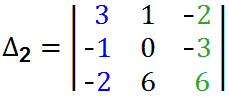= +3 (0 +18) -1[(-6)-(+6)] –2(-6-0)
= 54+12+12 = 78
2 = 78

= +3 (6 x 6) – (-3 x 0) – [-1(-1 x 6) – (-2 x 0)] + [1(-1) x (-3) – (-2) x (6)]
= 108 + 6 + 15
3 = 117

Now, solve and find the unknown values of current, i.e. i1, i2 and i3.
As, Cramer’s rule says that, variables i.e. i1 = ∆1/∆1, i2 = ∆/∆2 and i3 = ∆/∆3.
Therefore,

i1 = ∆1/∆1
= 117/39
i1 = 3A

And i2,
i2 = = ∆/∆2
= 78/39
i2 = 2A

And finally, i3;
i3 = ∆/∆3
= 117/39
i3 = 3A.

I hope that you understood the cramer’s rule very well and enjoyed the step by step tutorial. Please, don’t forget to share with your friends. Also, enter your email address in the below box to subscribe. So, we will send you more tutorials like the above one. Thanks.

Related Posts and Circuit Analysis Tools:

### Electrical Technology

1. henoke walie says:

it is very nice i like so macth

2. Arvind says:

How to find linear equation which is represent in form AX=B
where Matrix A in 4×2 Matrix(any Matrix).no other detail of B given

3. Vickey Gan says:

Nice and good

4. Roderick says:

Very nice.. thanks alot